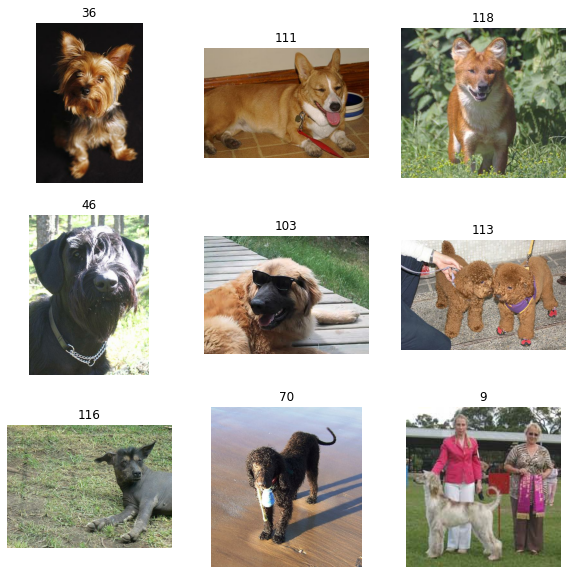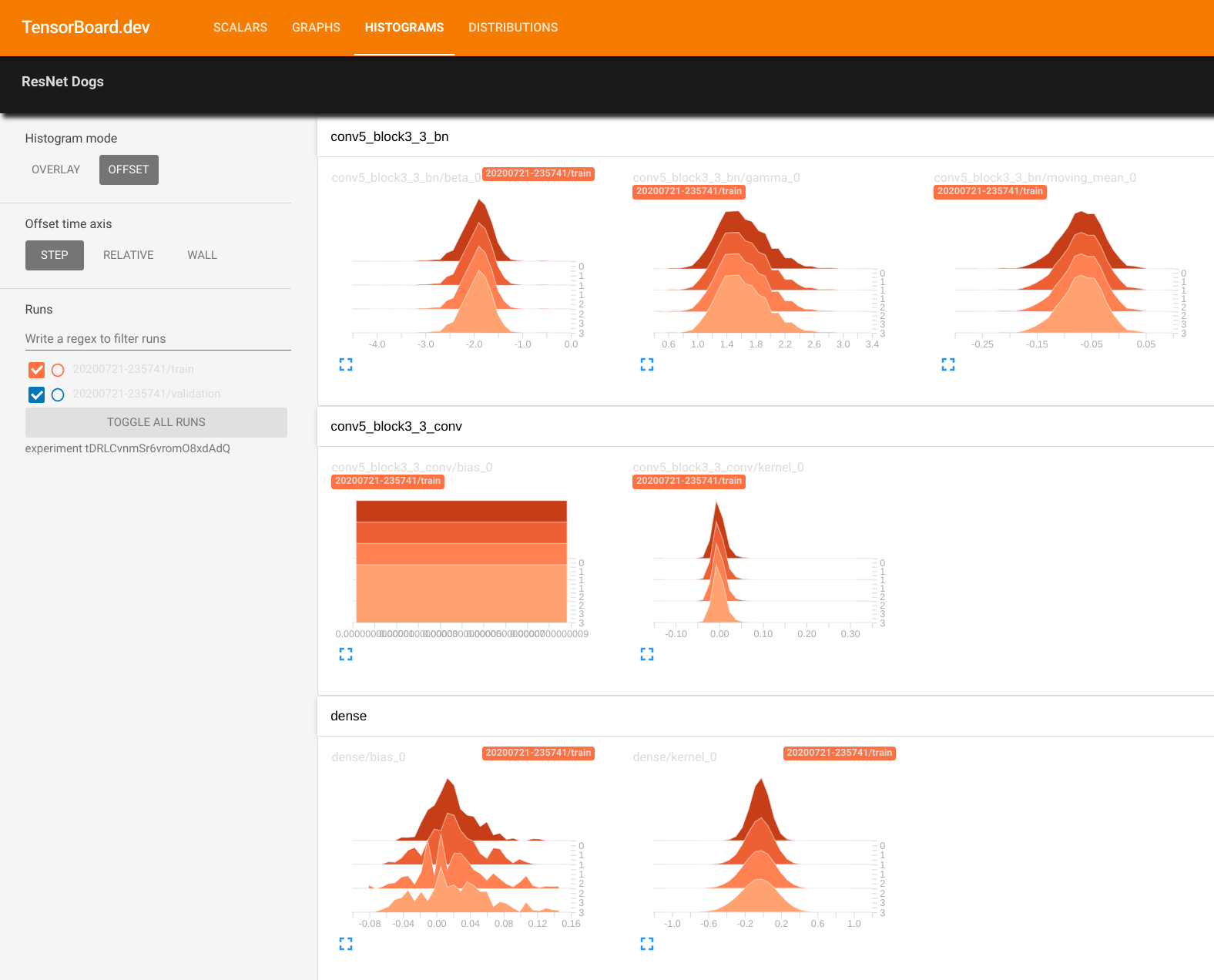TensorFlow Core ·August 03, 2020
Posted by Jonah Kohn and Pavithra Vijay, Software Engineers at Google

TensorFlow Cloud is a python package that provides APIs for a seamless transition from debugging and training your TensorFlow code in a local environment to distributed training in Google Cloud. It simplifies the process of training models on the cloud into a single, simple function call, requiring minimal setup and almost zero changes to your model. TensorFlow Cloud handles cloud-specific tasks such as creating VM instances and distribution strategies for your models automatically. This article demonstrates common use cases for TensorFlow Cloud, and a few best practices.We will walk through classifying dog breed images provided by the stanford_dogs dataset. To make this easy, we will use transfer learning with ResNet50 trained on ImageNet weights. Please find the code from this post here on the TensorFlow Cloud repository.

### Setup

Install TensorFlow Cloud using `pip install tensorflow_cloud`. Let’s start the python script for our classification task by adding the required imports.
``````import datetime
import os

import matplotlib.pyplot as plt
import numpy as np
import tensorflow as tf
import tensorflow_cloud as tfc
import tensorflow_datasets as tfds

from tensorflow import keras
from tensorflow.keras import layers
from tensorflow.keras.models import Model``````

TensorFlow Cloud runs your training job on Google Cloud using AI Platform services behind the scenes. If you are new to GCP, then please follow the setup steps in this section to create and configure your first Google Cloud Project. If you're new to using the Cloud, first-time setup and configuration will involve a little learning and work. The good news is that after the setup, you won’t need to make any changes to your TensorFlow code to run it on the cloud!
1. Create a GCP Project
2. Enable AI Platform Services
3. Create a Service Account
5. Create a Google Cloud Storage Bucket

#### GCP Project

A Google Cloud project includes a collection of cloud resources such as a set of users, a set of APIs, billing, authentication, and monitoring. To create a project, follow this guide. Run the commands in this section on your terminal.
``````export PROJECT_ID=<your-project-id>
gcloud config set project \$PROJECT_ID
``````

#### Service Account and Key

Create a service account for your new GCP project. A service account is an account used by an application or a virtual machine instance, and is used by Cloud applications to make authorized API calls.
``````export SA_NAME=<your-sa-name&rt;
gcloud iam service-accounts create \$SA_NAME
--member serviceAccount:\$SA_NAME@\$PROJECT_ID.iam.gserviceaccount.com \
--role 'roles/editor'``````
Next, we will need an authentication key for the service account. This authentication key is a means to ensure that only those authorized to work on your project will use your GCP resources. Create an authentication key as follows:
``gcloud iam service-accounts keys create ~/key.json --iam-account \$SA_NAME@\$PROJECT_ID.iam.gserviceaccount.com``
``export GOOGLE_APPLICATION_CREDENTIALS=~/key.json``

#### Cloud Storage Bucket

If you already have a designated storage bucket, enter your bucket name as shown below. Otherwise, create a Google Cloud storage bucket following this guide. TensorFlow Cloud uses Google Cloud Build for building and publishing a docker image, as well as for storing auxiliary data such as model checkpoints and training logs.
``GCP_BUCKET = "your-bucket-name"``

### Keras Model Creation

The model creation workflow for TensorFlow Cloud is identical to building and training a TF Keras model locally.

#### Resources

We'll begin by loading the stanford_dogs dataset for categorizing dog breeds. This is available as part of the tensorflow-datasets package. If you have a large dataset, we recommend that you host it on GCS for better performance.
``````(ds_train, ds_test), metadata = tfds.load(
"stanford_dogs",
split=["train", "test"],
shuffle_files=True,
with_info=True,
as_supervised=True,
)

Let's visualize the dataset:
``````print("Number of training samples: %d" % tf.data.experimental.cardinality(ds_train))
print("Number of test samples: %d" % tf.data.experimental.cardinality(ds_test))
print("Number of classes: %d" % NUM_CLASSES)``````
Number of training samples: 12000 Number of test samples: 8580 Number of classes: 120
``````plt.figure(figsize=(10, 10))
for i, (image, label) in enumerate(ds_train.take(9)):
ax = plt.subplot(3, 3, i + 1)
plt.imshow(image)
plt.title(int(label))
plt.axis("off")``````#### Preprocessing

We will resize and batch the data.
``````IMG_SIZE = 224
BATCH_SIZE = 64
BUFFER_SIZE = 2

size = (IMG_SIZE, IMG_SIZE)
ds_train = ds_train.map(lambda image, label: (tf.image.resize(image, size), label))
ds_test = ds_test.map(lambda image, label: (tf.image.resize(image, size), label))

def input_preprocess(image, label):
image = tf.keras.applications.resnet50.preprocess_input(image)
return image, label``````

#### Configure the input pipeline for performance

Now we will configure the input pipeline for performance. Note that we are using parallel calls and prefetching so that I/O doesn’t become blocking while your model is training. You can learn more about configuring input pipelines for performance in this guide.
``````ds_train = ds_train.map(
input_preprocess, num_parallel_calls=tf.data.experimental.AUTOTUNE
)

ds_train = ds_train.batch(batch_size=BATCH_SIZE, drop_remainder=True)
ds_train = ds_train.prefetch(tf.data.experimental.AUTOTUNE)

ds_test = ds_test.map(input_preprocess)
ds_test = ds_test.batch(batch_size=BATCH_SIZE, drop_remainder=True)``````

#### Build the model

We will be loading ResNet50 with weights trained on ImageNet, while using include_top=False in order to reshape the model for our task.
``````inputs = tf.keras.layers.Input(shape=(IMG_SIZE, IMG_SIZE, 3))
base_model = tf.keras.applications.ResNet50(
weights="imagenet", include_top=False, input_tensor=inputs
)
x = tf.keras.layers.GlobalAveragePooling2D()(base_model.output)
x = tf.keras.layers.Dropout(0.5)(x)
outputs = tf.keras.layers.Dense(NUM_CLASSES)(x)

model = tf.keras.Model(inputs, outputs)``````
We will freeze all layers in the base model at their current weights, allowing the additional layers we added to be trained.
``base_model.trainable = False``
Keras Callbacks can be used easily on TensorFlow Cloud as long as the storage destination is within your Cloud Storage Bucket. For this example, we will use the ModelCheckpoint callback to save the model at various stages of training, Tensorboard callback to visualize the model and its progress, and the Early Stopping callback to automatically determine the optimal number of epochs for training.
``````MODEL_PATH = "resnet-dogs"
checkpoint_path = os.path.join("gs://", GCP_BUCKET, MODEL_PATH, "save_at_{epoch}")
tensorboard_path = os.path.join(
"gs://", GCP_BUCKET, "logs", datetime.datetime.now().strftime("%Y%m%d-%H%M%S")
)
callbacks = [
tf.keras.callbacks.ModelCheckpoint(checkpoint_path),
tf.keras.callbacks.TensorBoard(log_dir=tensorboard_path, histogram_freq=1),
tf.keras.callbacks.EarlyStopping(monitor="val_loss", patience=3),
]``````

#### Compile the model

``````optimizer = tf.keras.optimizers.Adam(learning_rate=1e-2)
model.compile(
optimizer=optimizer,
loss=tf.keras.losses.SparseCategoricalCrossentropy(from_logits=True),
metrics=["accuracy"],
)``````

### Debug the model locally

We'll train the model in a local environment first in order to ensure that the code works properly before sending the job to GCP. We will use `tfc.remote()` to determine whether the code should be executed locally or on the cloud. Choosing a smaller number of epochs than intended for the full training job will help verify that the model is working properly without overloading your local machine.
``````if tfc.remote():
epochs = 500
train_data = ds_train
test_data = ds_test
else:
epochs = 1
train_data = ds_train.take(5)
test_data = ds_test.take(5)
callbacks = None

model.fit(
train_data, epochs=epochs, callbacks=callbacks, validation_data=test_data, verbose=2
)``````
``````if tfc.remote():
SAVE_PATH = os.path.join("gs://", GCP_BUCKET, MODEL_PATH)
model.save(SAVE_PATH)``````

### Model Training on Google Cloud

To train on GCP, populate the example code with your GCP project settings, then simply call `tfc.run()` from within your code. The API is simple with intelligent defaults for all the parameters. Again, we don’t need to worry about cloud specific tasks such as creating VM instances and distribution strategies when using TensorFlow Cloud. In order, the API will:
• Convert it into a docker image with required dependencies.
• Run the training job on a GCP cluster.
• Stream relevant logs and store checkpoints.
The `run()` API provides significant flexibility for use, such as giving users the ability to specify custom cluster configuration, custom docker images. For a full list of parameters that can be used to call `run()`, see the TensorFlow Cloud readme.

Create a `requirements.txt` file with a list of python packages that your model depends on. By default, TensorFlow Cloud includes `TensorFlow` and its dependencies as part of the default docker image, so there's no need to include these. Please create `requirements.txt` in the same directory as your python file. `requirements.txt` contents for this example are:
``````tensorflow-datasets
matplotlib``````
By default, the `run` API takes care of wrapping your model code in a TensorFlow distribution strategy based on the cluster configuration you have provided. In this example, we are using a single node multi-gpu configuration. So, your model code will be wrapped in a TensorFlow `MirroredStrategy` instance automatically.

Call `run()` in order to begin training on cloud. Once your job has been submitted, you will be provided a link to the cloud job. To monitor the training logs, follow the link and select ‘View logs’ to view the training progress information.
``````tfc.run(
requirements_txt="requirements.txt",
distribution_strategy="auto",
chief_config=tfc.MachineConfig(
cpu_cores=8,
memory=30,
accelerator_type=tfc.AcceleratorType.NVIDIA_TESLA_T4,
accelerator_count=2,
),
docker_image_bucket_name=GCP_BUCKET,
)``````

#### Visualize the model using TensorBoard

Here, we are loading the Tensorboard logs from our GCS bucket to evaluate model performance and history.
``tensorboard dev upload --logdir "gs://your-bucket-name/logs" --name "ResNet Dogs"``#### Evaluate the model

After training, we can load the model that's been stored in our GCS bucket, and evaluate its performance.
``````if tfc.remote():